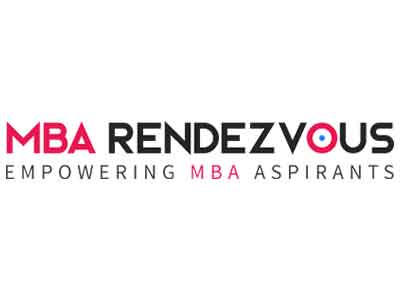# CAT 2013 Preparation Alert: Maximum weightage is given to Geometry in QACAT Notification: August 2023CAT 2013 will be conducted from 16th October to 11th November and notification to this effect is likely to be out on July 21, 2013 (Watch this space for latest news on CAT 2013)

CAT 2013 aspirants may note that  maximum weightage is given to geometry, although every CAT paper will have 3-4 questions on mensuration, as well as a couple of questions on coordinate geometry, totaling about 25-30% of questions in the QA section.

Topics that need to be covered in geometry are basic theorems involving triangles, circles and parallel lines.

A common type of question that is often asked in CAT is to find the value of certain angles or length of certain sides. Therefore, make sure that you cover topics such as congruency and similarity of triangles.

In coordinate geometry important is that you need to practice on straight lines and circles. Given the equation of a circle, you should be able to comment on the centre and radius of the circle and draw it on a piece of graph paper.

Similarly, you should know what the slope and y-intercept of a given straight line equation is, and be able to draw the line on a piece of graph paper.

For mensuration, flip through a school level textbook for basic formulae on areas, surface areas and volumes of triangles, circles, cylinders, cones, cuboids and spheres. Mensuration problems are calculation intensive, and require lots of practice.

Stay informed and inspired at MBA Rendezvous